• 单因素试验固定效应模型方差分析 观测值的线性模型 平方和与自由度分解 例题与SPSS求解 非平衡单因素试验SPSS求解 一、观测值的线性模型 单因素试验线性可加模型为： Yij为第i个处理的第j个观测值；U为所有观测值...
单因素试验固定效应模型方差分析

观测值的线性模型
平方和与自由度分解
例题与SPSS求解
非平衡单因素试验SPSS求解

一、观测值的线性模型
单因素试验线性可加模型为：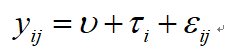Yij为第i个处理的第j个观测值；U为所有观测值的平均值；Ti为第i个处理效应；Eij为随机误差。
二、平方和与自由度分解
平凡和的分解这里不做介绍，因为单因素方差分析大部分统计软件都能够计算，没有必要进行手动计算；另外还有一个原因就是CSDN编辑公式比较麻烦，这里就不敲了。PS：如果大家在CSDN有好的公式展示方法，后面会补上！~~目前需要的童鞋，自行查询相应书籍。
自由度分解：
自由度分解比较简单，下面详细介绍一下，大家可以根据自由度判断使用的软件给出的方差分析表是否正确，这是一个比较快速的方法。
如果一个单因素试验，一共有a个处理，每个处理重复n次，则一共有an个观测值，具有一个约束条件所有的观测值减去观测平均值后，求和结果为0，因此总自由度为an-1；a个处理之间，每个处理观测值的平均值，和所有观测平均值之差求和为0，所以处理间自由度为a-1；而每个处理内部，n个观测值与该处理下观测平均值之差求和为0，一共有a个处理，则处理内自由度为a*（n-1）。
总的来说，就是总自由度为观测值个数减一，也就是an-1；处理间自由度为处理个数减一，a-1；处理内自由度为a（n-1）。
三、例题与SPSS求解
某个树种5个不同种源种子的重量比较，分别称取100粒不同种源种子的重量，每个种源称量8次（每一批种子不同），观测值如下：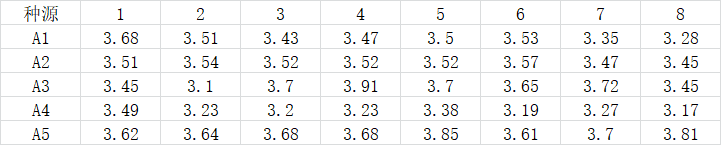SPSS求解：
SPSS数据表格如下：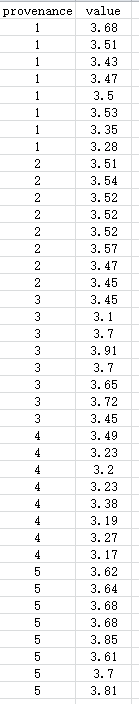软件操作：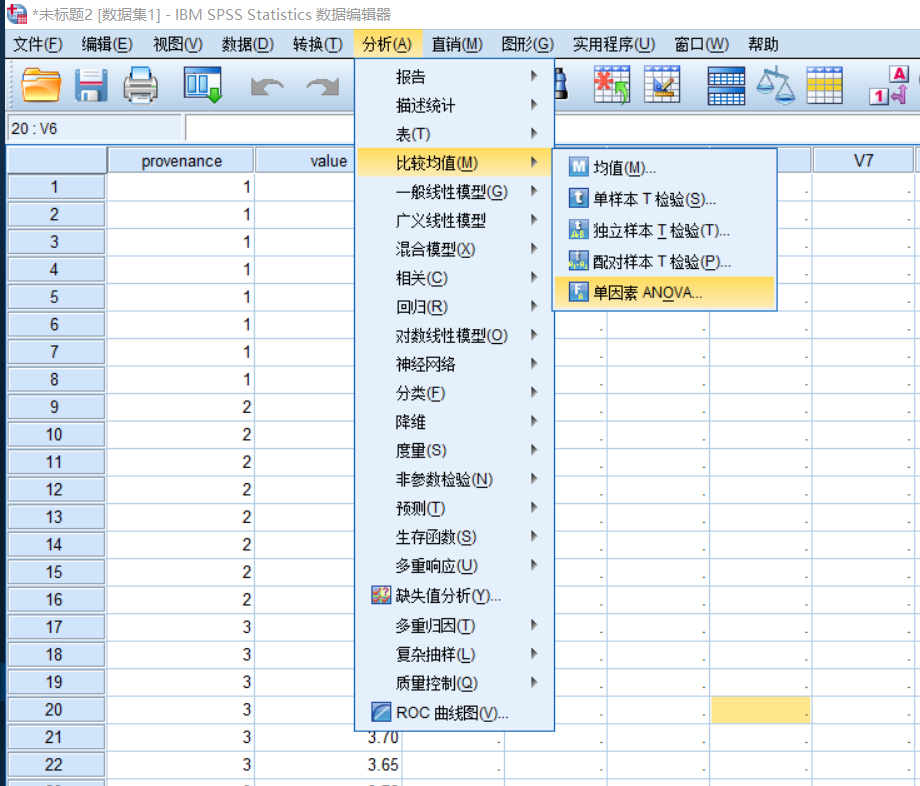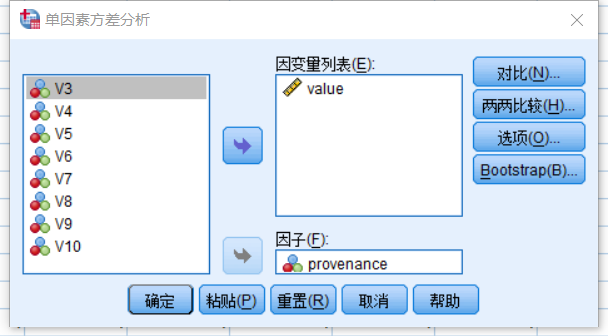结果：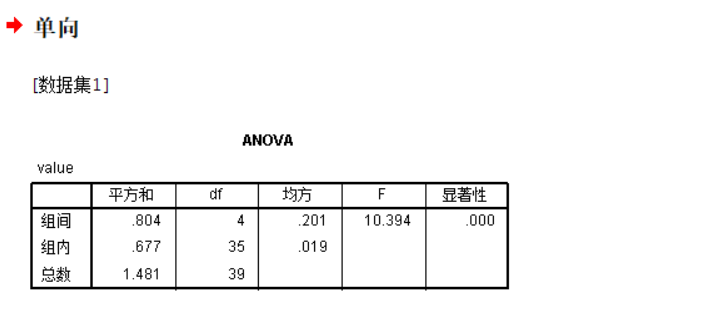组间显著性小于0.000，说明种源种子质量之间差异极显著。
下一章会介绍利用SPSS进行单因素非平衡试验方差分析，以及多重对比


展开全文方差分析
• 平均差值推论处理的作用。 随机区组设计：处理只能在区组内随机分配，每个实验单位接受的处理是不相同的；要求每个区组内实验单位彼此独立。 重复测量设计：“处理”是在区组（受试者）间随机分配，区组内的各...
• 其次，为了描述财务时间序列的高峰和肥尾以及杠杆效应，本文使用基于自回归综合移动平均模型的偏斜非对称幂自回归条件异方差模型来分析销售数据。 实证分析表明，考虑偏态分布的模型是有效的。
• 除此之外，SEM还能评估构面是否具有信效度、处理复杂的模型或理论（如，模型中有中介、调节效应等）、有效率的处理Missing Data（SEM的多重插补法比用平均值取代缺失值更有优势）；最后，使用Mplus跑结构方程模型的...
为什么要用结构方程模型（SEM）呢？首先，由于心理测量的构面不可能不受到测量误差的影响，SEM能处理测量误差；除此之外，SEM还能评估构面是否具有信效度、处理复杂的模型或理论（如，模型中有中介、调节效应等）、有效率的处理Missing Data（SEM的多重插补法比用平均值取代缺失值更有优势）；最后，使用Mplus跑结构方程模型的话，Mplus软件独特的估计方法（WLSMV）能够处理类别或顺序数据，如李克特量表少于5点时可处理。综上所述，SEM的多个优点真的让我们无法抗拒它。既然SEM优点这么多，那么怎么用Mplus构建模型呢？我先来介绍一下SEM常用的名词，关于语法，请留意下一次推送哦~大家来看一下这个模型1、参数（parameter）：又叫做母数，带有未知与估计的特质，在无说明情况下，默认为自由参数。2、自由参数（free parameter）：在Mplus所画的每一条线均是一个参数，除设为固定参数外，自由估计的参数越多，自由度（df）越小。3、固定参数（fix/constrain parameter）：Mplus图上被设定为0或1或任何数字的线。4、观察变量（item/indicator/observed/measured/ manifest variable）：数据文件中的变量，一般可以直接观察，并进行测量的变量，如年龄、体重、身高等。5、潜在变量（dimension/latent/unobserved variable/ factor/ construct）：数据文件中无法显示，无法进行测量，由观察变量反映的变量，如信任、心理特性等。⋆潜变量用来解释观察变量，潜变量之间“因果关系”或“相关关系”则为研究的假设。6、误差（Error/E）：测量变量被估计后无法解释的方差，模型中e1-e6。7、干扰（Disturbance/D）：潜变量经估计后无法解释的方差，模型中e7。8、外生变量（Exogenous）：用来观测变量的变量，本身不具有测量误差，分为外生潜在变量及外生观察变量，可理解为自变量。模型中没有被任何箭头指向的变量，F3。9、内生变量（endogenous）：被自变量预测的变量，本身具有测量误差的变量，其自身也可以去预测的变量，分为内生潜在变量和内生观察变量。模型中被箭头指向的变量，F1-F2、Q1-Q3、S1-S3。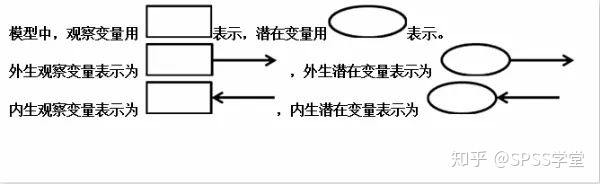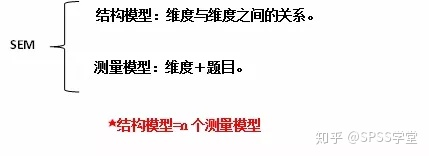展开全文• ## DID模型

千次阅读 2020-05-18 22:02:37
政策的实施有滞后效应，因此需要把政策的实施变量dst滞后几期验证政策实施的平均效应。 实现DID需要共同趋势检验方法： 如果实验组与对照组不满足共同趋势假定，需如下处理： 1、加权对照...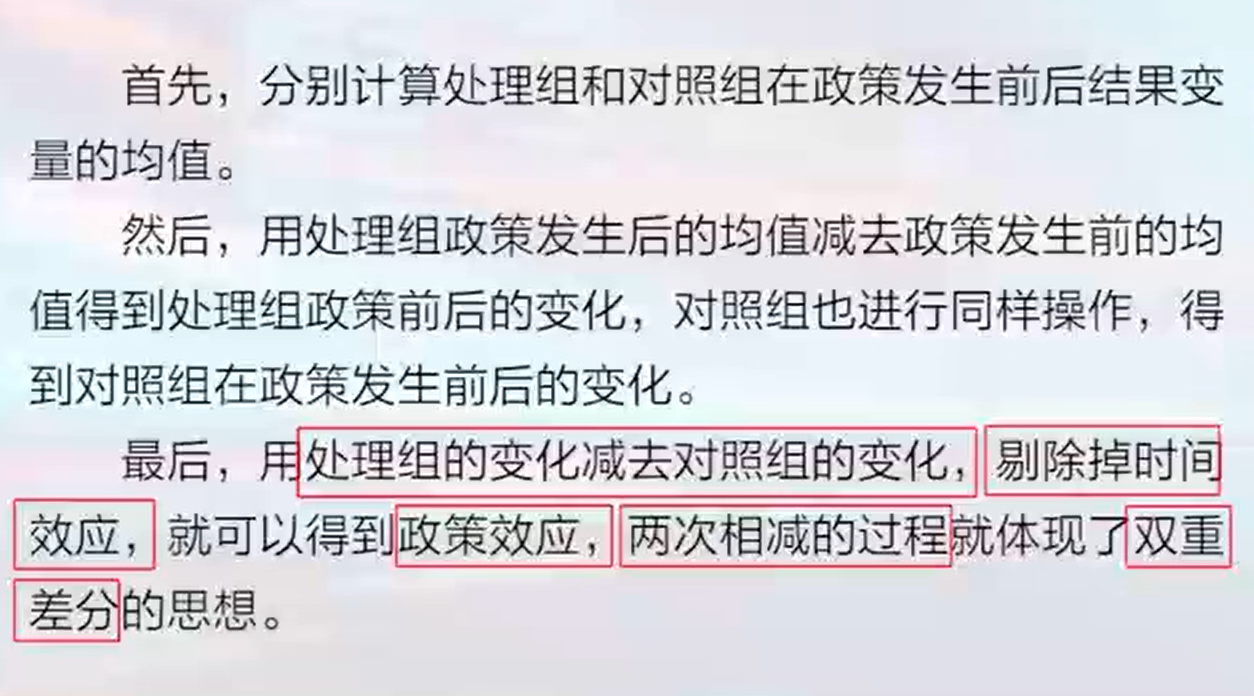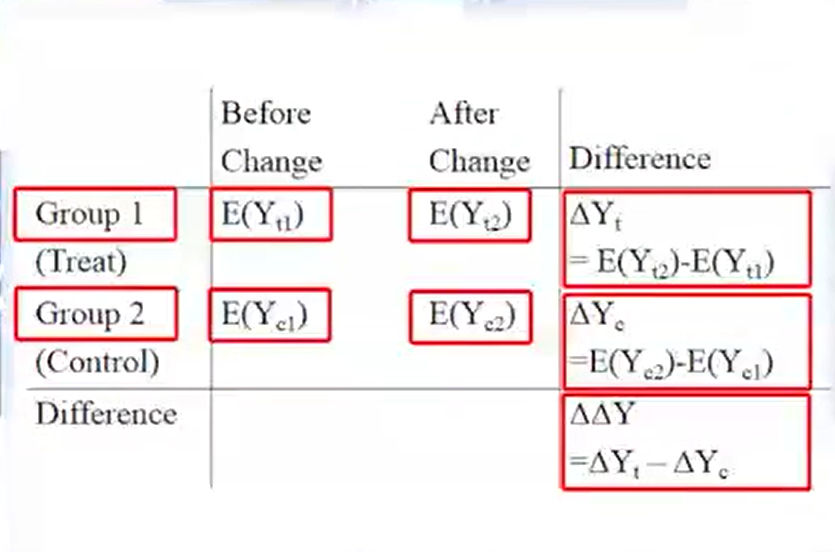E(yt1)E(yc1):政策变化前结果的均值。E(yt2)E(yc2):政策变化后结果的均值 delta(yt)反应实验组政策变化后的结果变化，delta（yc）反应control组证的变化前后的差。delta(delta(y))即是政策产生的影响。

did前提：共同趋势假定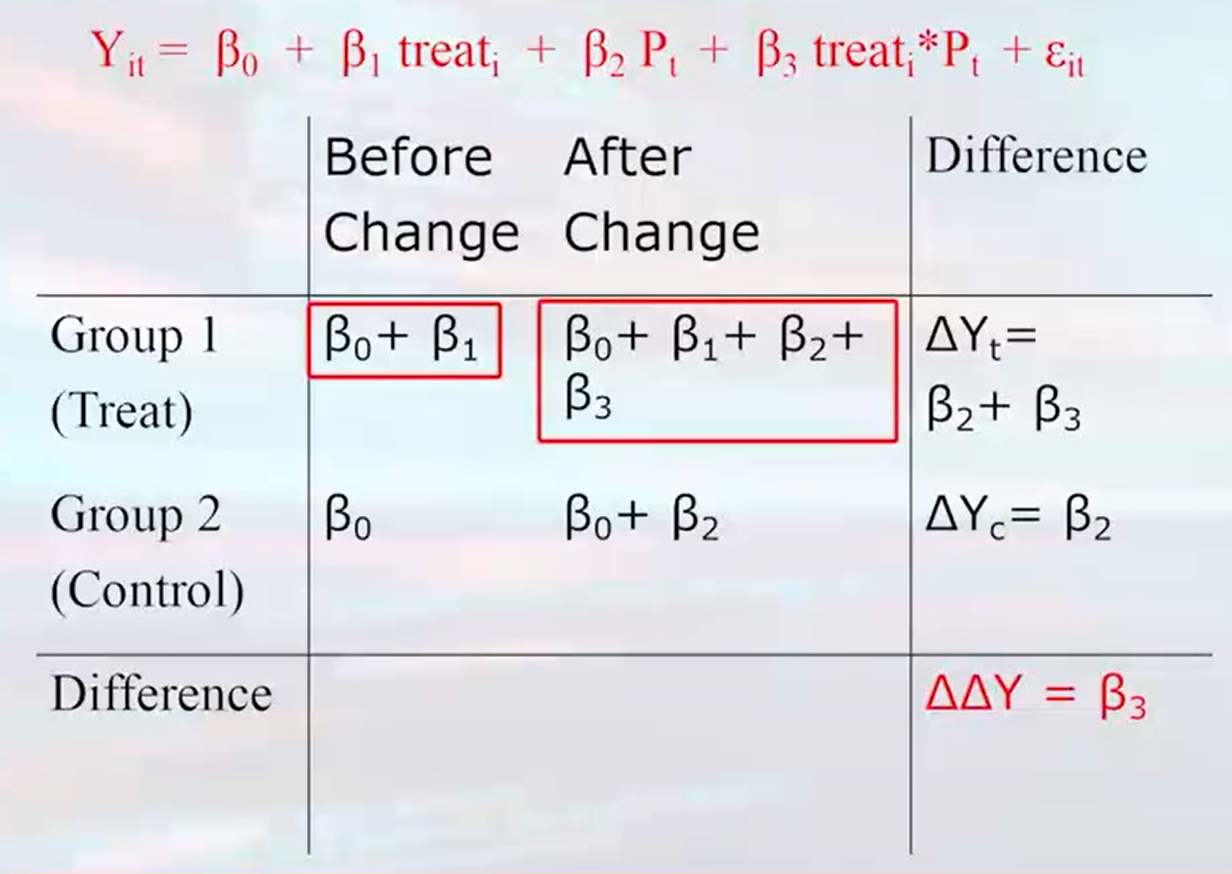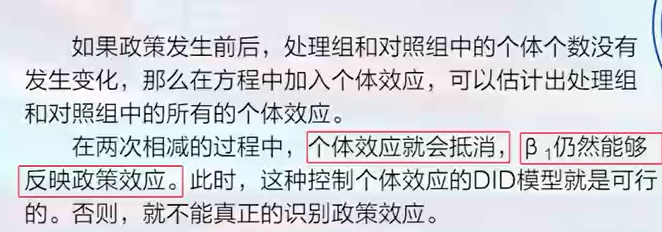政策的实施有滞后效应，因此需要把政策的实施变量dst滞后几期验证政策实施的平均效应。

实现DID需要共同趋势检验方法：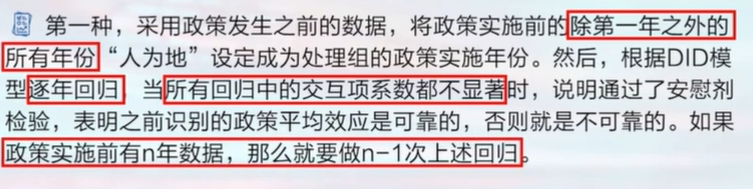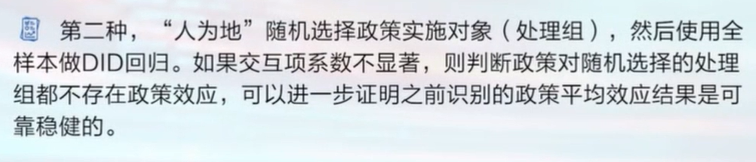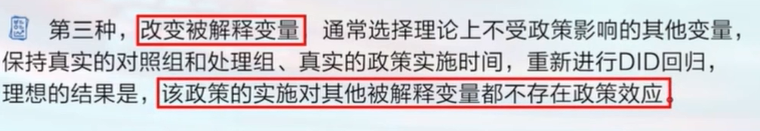如果实验组与对照组不满足共同趋势假定，需如下处理：

1、加权对照组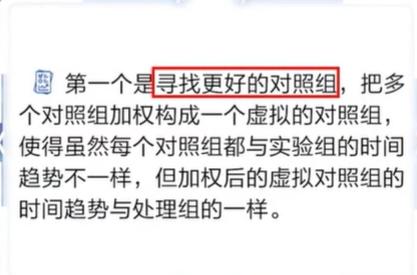2、合成控制法

3、时间趋势法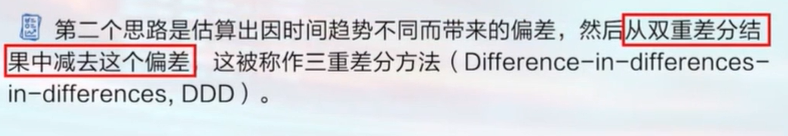展开全文• 模型特性： 所有卷积层都是使用ReLU 作为非线性映射函数，使模型收敛速度更快 在多个GPU 上进行模型的训练，不但可以提高模型的训练速度，还能提升数据的使用规模 ... s, 避免平均池化的平均效应...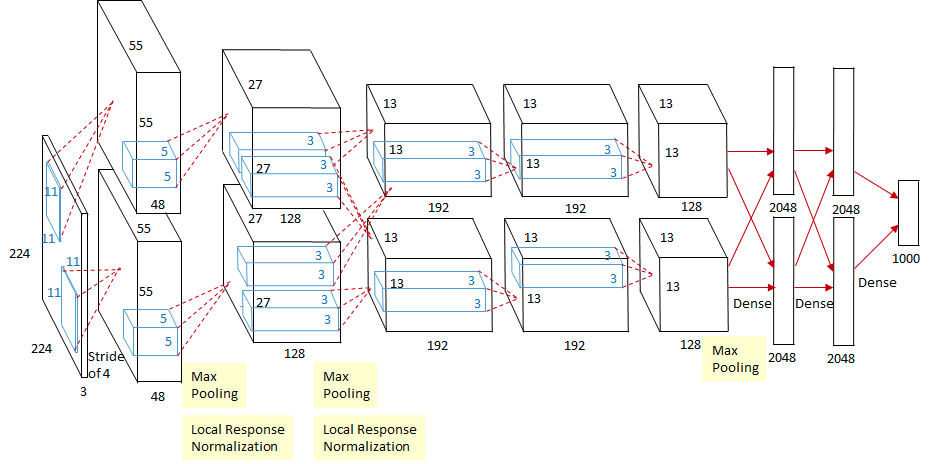模型特性：

所有卷积层都是使用ReLU 作为非线性映射函数，使模型收敛速度更快
在多个GPU 上进行模型的训练，不但可以提高模型的训练速度，还能提升数据的使用规模
使用 dropout 而不是正则化来处理过度拟合。当然，这也导致了训练时间加长。
重叠最大池化（overlapping max pooling) , 即池化范围 z 与步长 s 存在关系  z > s, 避免平均池化的平均效应。
使用 LRN 对局部特征进行归一化，增强了模型的泛化能力

AlexNet是由 Alex Krizhevsky 提出的首个应用于图像分类的深层卷积神经网络，该网络在2012年ILSVRC（ImageNet Large Scale Visual Recognition Competition）图像分类竞赛中以15.3%的top-5测试错误率赢得第一名。AlexNet使用GPU代替CPU进行运算，使得在可接受的时间范围内模型结构能够更加复杂，它的出现证明了深层卷积神经网络在复杂模型下的有效性。
LRN (Local Response Normalization) :   局部特征归一化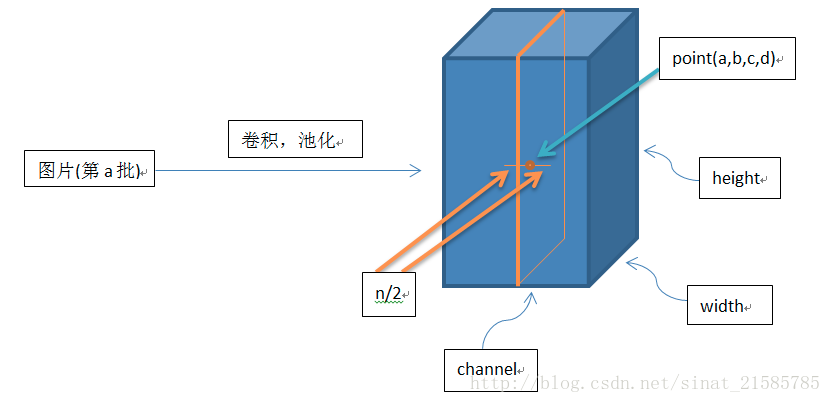公式：
$b_{x, y}^{i}=a_{x, y}^{i} /\left(k+\alpha \sum_{j=\max (0, i-n / 2)}^{\min (N-1, i+n / 2)}\left(a_{x, y}^{j}\right)^{2}\right)^{\beta}$
主要 $a_{x, y}^{i}$ 为  [a, b, c, d] = [batch_size, height, width, channel]  看图解释。
sqr_sum[a, b, c, d] =
sum(input[a, b, c, d - depth_radius : d + depth_radius + 1] ** 2)
output = input / (bias + alpha * sqr_sum) ** beta

tensorflow ：lrn参数解释：
def lrn(input, depth_radius=5, bias=1, alpha=1, beta=0.5, name=None):
r"""Local Response Normalization.

The 4-D input tensor is treated as a 3-D array of 1-D vectors (along the last
dimension), and each vector is normalized independently.  Within a given vector,
each component is divided by the weighted, squared sum of inputs within
depth_radius.  In detail,

sqr_sum[a, b, c, d] =
sum(input[a, b, c, d - depth_radius : d + depth_radius + 1] ** 2)
output = input / (bias + alpha * sqr_sum) ** beta

For details, see [Krizhevsky et al., ImageNet classification with deep
convolutional neural networks (NIPS 2012)](http://papers.nips.cc/paper/4824-imagenet-classification-with-deep-convolutional-neural-networks).

Args:
input: A Tensor. Must be one of the following types: half, bfloat16, float32.
4-D.
depth_radius: An optional int. Defaults to 5.
0-D.  Half-width of the 1-D normalization window.
bias: An optional float. Defaults to 1.
An offset (usually positive to avoid dividing by 0).
alpha: An optional float. Defaults to 1.
A scale factor, usually positive.
beta: An optional float. Defaults to 0.5. An exponent.
name: A name for the operation (optional).

Returns:
A Tensor. Has the same type as input.
"""

这里解释为什么 输入图片大小建议为 $227\times 227$
首先由计算公式

$output = \frac{i - k}{s} + 1$

SAME 模式 加入 padding 之后， 输出长度或者宽度 与输入的长度或宽度在 stride 下保持一致：

$output = \frac{i}{s}$
​假设左右两边需要补的总 padding 为 $P_a$ 那么：
$output = \frac{i-k+P_a}{s} + 1$
​				反过来计算：
$P_a = (output - 1) \times s + k - i$
​				左边需要补 padding 为 $P_l = \frac{P_a}{2}$, 右边需要补padding 为 $P_r = P_a - P_l$ 即 $P_r = \frac{P_a}{2}$
​				整体公式可为:
$output = \frac {(i-k+2p)}{s} + 1$
AlexNet 的第二层 输出维度为  $55 \times 55$
步长stride：  s = 4
卷积核 kernel (filter) 大小 :    k = $11 \times 11$
假设 输入 为：   i = $224 \times 224$
如果是 224 的话，会出现小数，当计算 $P_r$ 时，
$P_a = (output - 1) \times s + k - i = (55 - 1）\times 4 + 11 - 224 = 3$
$P_r = \frac{3}{2} = 1.5 (向上取整) = 2$
$output = \frac{(i-k+2p)}{s} + 1 = \frac{(224 - 11 + 2 * 2)}{4} + 1 = 55.25$
上式会出现 55.25 出现小数点。不方便处理后续调整
如果是 227 的话
$P_a = (55 - 1) \times 4 + 11 - 227 = 0$
把 AlexNet 解析成一个 GPU 来处理
使用一个GPU时，把他们串联，把 输入224 替换为 227

Size / Operation
Filter
Depth
Stride
Number of Parameters
Forward Computation

3* 227 * 227

Conv1 + Relu
11 * 11
96
4

(11 * 11 * 3 + 1) * 96=34944
(11 * 11 * 3 + 1) * 96 * 55 * 55=105705600

96 * 55 * 55

Max Pooling
3 * 3

2

96 * 27 * 27

Norm

Conv2 + Relu
5 * 5
256
1
2
(5 * 5 * 96 + 1) * 256=614656
(5 * 5 * 96 + 1) * 256 * 27 * 27=448084224

256 * 27 * 27

Max Pooling
3 * 3

2

256 * 13 * 13

Norm

Conv3 + Relu
3 * 3
384
1
1
(3 * 3 * 256 + 1) * 384=885120
(3 * 3 * 256 + 1) * 384 * 13 * 13=149585280

384 * 13 * 13

Conv4 + Relu
3 * 3
384
1
1
(3 * 3 * 384 + 1) * 384=1327488
(3 * 3 * 384 + 1) * 384 * 13 * 13=224345472

384 * 13 * 13

Conv5 + Relu
3 * 3
256
1
1
(3 * 3 * 384 + 1) * 256=884992
(3 * 3 * 384 + 1) * 256 * 13 * 13=149563648

256 * 13 * 13

Max Pooling
3 * 3

2

256 * 6 * 6

Dropout (rate 0.5)

FC6 + Relu

256 * 6 * 6 * 4096=37748736
256 * 6 * 6 * 4096=37748736

4096

Dropout (rate 0.5)

FC7 + Relu

4096 * 4096=16777216
4096 * 4096=16777216

4096

FC8 + Relu

4096 * 1000=4096000
4096 * 1000=4096000

1000 classes

Overall

62369152=62.3 million
1135906176=1.1 billion

Conv VS FC

Conv:3.7million (6%) , FC: 58.6 million  (94% )
Conv: 1.08 billion (95%) , FC: 58.6 million (5%)

TensorFlow 代码实现：
先解释Conv ，  fc 等辅助函数：

计算卷积 Conv + ReLU:

# Conv RelU
def conv(x
, filter_height
, filter_width
, num_filters
, stride_y
, stride_x
, name

# 输入通道数量
input_channels = int(x.get_shape()[-1])

# 利用 tf.nn.conv2d 卷积计算
convolve = lambda i, k: tf.nn.conv2d(i, k,
strides = [1, stride_y, stride_x, 1],

with tf.variable_scope(name) as scope:
# 创建 权重和偏差值
weights = tf.get_variable('weights',
shape = [filter_height, filter_width,
input_channels/groups, num_filters])

biases = tf.get_variable('biases', shape=[num_filters])

if groups == 1:
conv = convolve(x, weights)
# 在多个组的情况下，拆分输入和权重
else:
# 拆分输入和权重并分别对其进行卷积
input_groups = tf.split(axis = 3, num_or_size_splits=groups, value=x)
weight_groups = tf.split(axis = 3, num_or_size_splits=groups, value=weights)
output_groups = [convolve(i, k) for i,k in zip(input_groups, weight_groups)]
conv = tf.concat(axis = 3, values = output_groups)

relu = tf.nn.relu(bias, name=scope.name)
return relu


计算 最大池化层

def max_pool(x, filter_height, filter_width, stride_y, stride_x
return tf.nn.max_pool(x, ksize=[1, filter_height, filter_width, 1]
, strides = [1, stride_y, stride_x, 1],


计算 局部特征归一化 LRN（Local Response Normalization）

def lrn(x, radius, alpha, beta, name, bias=1.0):
alpha = alpha, beta = beta,
bias = bias, name = name)


计算 dropout 正则化

def dropout(x, keep_prob):
return tf.nn.dropout(x, keep_prob)


计算全连接层 fc + ReLU

def fc(x, num_in, num_out, name, relu=True):
with tf.variable_scope(name) as scope:
weights = tf.get_variable('weights', shape=[num_in, num_out], trainable=True)
biases = tf.get_variable('biases', [num_out], trainable=True)

act = tf.nn.xw_plus_b(x, weights, biases, name=scope.name)

if relu == True:
relu = tf.nn.relu(act)
return relu
else:
return act


TensorFlow 实现AlexNet 网络,全部代码，github地址:  alexnet.py

import tensorflow as tf

def AlexNet(x, keep_prob, num_classes):
# 第一层: Conv (w ReLu) -> Lrn -> Pool
conv1 = conv(x, 11, 11, 96, 4, 4, padding='VALID', name='conv1')
norm1 = lrn(conv1, 2, 2e-05, 0.75, name='norm1')
pool1 = max_pool(norm1, 3, 3, 2, 2, padding='VALID', name='pool1')

# 第二层: Conv (w ReLu)  -> Lrn -> Pool with 2 groups
conv2 = conv(pool1, 5, 5, 256, 1, 1, groups=2, name='conv2')
norm2 = lrn(conv2, 2, 2e-05, 0.75, name='norm2')
pool2 = max_pool(norm2, 3, 3, 2, 2, padding='VALID', name='pool2')

# 第三层: Conv (w ReLu)
conv3 = conv(pool2, 3, 3, 384, 1, 1, name='conv3')

# 第四层: Conv (w ReLu)
conv4 = conv(conv3, 3, 3, 384, 1, 1, groups=2, name='conv4')

# 第五层: Conv (w ReLu) -> Pool 拆分两组
conv5 = conv(conv4, 3, 3, 256, 1, 1, groups=2, name='conv5')
pool5 = max_pool(conv5, 3, 3, 2, 2, padding='VALID', name='pool5')

# 第六层: Flatten -> FC (w ReLu) -> Dropout
flattened = tf.reshape(pool5, [-1, 6*6*256])
fc6 = fc(flattened, 6*6*256, 4096, name='fc6')
dropout6 = dropout(fc6, keep_prob)

# 第七层: FC (w ReLu) -> Dropout
fc7 = fc(dropout6, 4096, 4096, name='fc7')
dropout7 = dropout(fc7, keep_prob)

# 第八层全连接层没有 relu激活层: FC
fc8 = fc(dropout7, 4096, num_classes, relu = False, name='fc8')
return fc8

def conv(x, filter_height, filter_width, num_filters, stride_y, stride_x, name,
# 输入通道数数量
input_channels = int(x.get_shape()[-1])

# 创建权重和偏差值
with tf.variable_scope(name) as scope:
weights = tf.get_variable('weights', shape=[filter_height,
filter_width,
input_channels/groups,
num_filters])
biases = tf.get_variable('biases', shape=[num_filters])

if groups == 1:
conv = tf.nn.conv2d(x, weights,
strides=[1, stride_y, stride_x, 1],
# 在有多组的情况下，拆分输入权重
else:
# 拆分输入和权重并进行卷积
input_x = tf.split(axis=3, num_or_size_splits=groups, value=x)
weight_w = tf.split(axis=3, num_or_size_splits=groups,
value=weights)
output_groups = []
for i, k in zip(input_x, weight_w):
output_conv2d = tf.nn.conv2d(i, k,
strides=[1, stride_y, stride_x, 1],
output_groups.append(output_conv2d)
# 创建连接
conv = tf.concat(axis=3, values=output_groups)

# 偏差值

# 激活函数Relu
relu = tf.nn.relu(bias, name=scope.name)
return relu

# 创建全连接层
def fc(x, num_in, num_out, name, relu=True):
with tf.variable_scope(name) as scope:
# 创建权重和偏差值
weights = tf.get_variable('weights', shape=[num_in, num_out],
trainable=True)
biases = tf.get_variable('biases', [num_out], trainable=True)

# 计算多个输入，权重，偏差值
act = tf.nn.xw_plus_b(x, weights, biases, name=scope.name)
if relu == True:
return tf.nn.relu(act)
else:
return act

# 创建max_pool最大池化层
def max_pool(x, filter_height, filter_width, stride_y, stride_x, name,
return tf.nn.max_pool(x, ksize=[1, filter_height, filter_width, 1],
strides=[1, stride_y, stride_x, 1],

# 创建 LRN 层
def lrn(x, radius, alpha, beta, name, bias=1.0):
alpha=alpha, beta=beta,
bias=bias, name=name)

# 创建dropout层
def dropout(x, keep_prob):
return tf.nn.dropout(x, rate=1-keep_prob)


具体训练github 的地址: https://github.com/BillyLearn/paper/tree/master/tensorflow_alexnet
训练： python train.py
图片测试： jupyter notebook 运行 image_validate.ipynb
参考资料：
深度学习500问经典网络 AlexNet模型
论文AlexNet
深度学习局部响应归一化LRN
python - What is the difference between ‘SAME’ and ‘VALID’ padding in tf.nn.max_pool of tensorflow? - Stack Overflow
alexnet-with-tensorflow


展开全文AlexNet TensorFlow
• 使用SAS的PROC MIXED程序通过混合模型进行统计分析，该模型包括将动物作为随机效应，将治疗作为固定效应（通过协变量调整）。 第一天奶牛平均产奶量为23.7（±2.0）kg，两种处理之间无差异（P <0.96）。 与C...
• 用离子束溅射技术和热处理方法,制备出颗粒尺寸和镶嵌密度均可控制的高质量Ge-SiO2纳米颗粒镶嵌薄膜。在室温下测量了不同粒度纳米锗...用有效质量近似模型讨论了量子尺寸效应和介电限域效应对纳米锗颗粒电子结构的影响。
• 介子系统的动力学使用通用的退相干模型进行处理，并考虑了系统的衰减特性。 在最近在LSND（液体闪烁体中微子检测器）实验中使用的去相干模型框架内，在三种风味振荡的背景下研究了中微子动力学。 对于介子系统，去...
• 提出并构建了一个改善因子模型来描述设备维护的改善效果,该模型综合考虑了维护成本、设备年龄以及维护过程学习效应等影响因素.考虑两组件间故障率存在交互作用且随着交互...
• 这些时空被认为是多维全局单极子（如果n⩾3）或宇宙弦（如果n = 2）具有（d-n-1）平坦额外维度的简单模型。 因此，我们称它们为圆锥形背景。 根据角度赤字值，我们推导出标量Green函数的扰动表达式，该表达式对于...
• 因此，如何改进这些图像的质量，就成为数字图像处理中的～个重要任务。本论文对基于小波变换的图像去噪方法进行了研究，主要包括以内容： 1．门限法小波图像去噪研究 小波门限法是一种经典的小波去噪方法。由于小波...
• 在没有其他外部姿态参考可用的情况下，对于典型的磁罗盘的直接航向角估计，提出了两步优化校正算法，以校正由附近的硬铁和软铁引起的模型系数。 具体而言，在步骤1中，使用具有椭圆体约束的Levenberg-Marquardt（LM...研究论文
• 　数值变量资料的一般分析：参数估计，ｔ检验，单因素和多因素的方差分析，协方差分析，交互效应模型，平衡和非平衡设计，嵌套设计，随机效应，多个均数的两两比较，缺项数据的处理，方差齐性检验，正态性检验，变量...
• 性能评价指标是客观评价信息系统性能的依据，一般包括系统平均无故障时间，系统联机响应时间、处理速度、吞吐量、操作灵活性，系统加工数据的准确性，系统的可扩充性等。 业务流程分析： 业务流程分析的目的是为了...
• 10.2 滑动平均FIR滤波器 10.3 梳状FIR滤波器 10.4 L波段FIR滤波器 10.5 镜像FIR滤波器 10.6 补码FIR滤波器 10.7 频率抽样滤波器组 10.8 卷积平滑FIR滤波器 10.9 非线性相位FIR滤波器 10.10 Farrow FIR滤波器 第11章 ...
• 双线性插值利用源像素点周围邻近的几个像素点的线性平均权值来计算目标像素点值,具有一定的边缘平滑作用,但会使图像的细节产生退化,丢失重要的边缘特征;三次样条插值放缩后的图像具有较高的光滑性,但计算量大、且易...matlab 插值
• 　10.2滑动平均FIR滤波器 　10.3梳状FIR滤波器  10.4 L波段FIR滤波器 　10.5镜像FIR滤波器 　10.6补码FIR滤波器 　10.7频率抽样滤波器组 　10.8卷积平滑FIR滤波器 　10.9非线性相位FIR滤波器 　10.10Farrow FIR...
• 33. 4 多径信道：远近效应和功率控制 33. 5 多径：瑞克接收机和干扰抵消 33. 6 干扰抵消：非线性多用户检测器 33. 7 近似 33. 8 中断率 33. 9 示例 参考文献 附录A 匹配前端处理器输出的相关函数 A. 1 慢变化信道...
• 第七章：信号的运算和处理 （1） 掌握比例、加减、积分、微分、对数和指数电路的工作原理及运算关系，能够运用“虚短”和“虚断”的概念分析各种运算电路输出电压与输入电压之间的运算关系，能够根据需要合理地选择...
• 9.2.3　加载后自动计算平均法向量　286 9.2.4　加载纹理坐标　289 9.3　本章小结　292 第10章　混合与雾　293 10.1　混合技术　293 10.1.1　混合基本知识　293 10.1.2　源因子和目标因子　294 10.1.3　简单...
• 为了建立一个具有QoS能力的Internet，网络的设计者必须开发和配置新型的路由器，这些路由器能够将分组划分成不同的类型，在网络拥塞的时候对不同类别的分组分别进行排队，并为每一类别分配唯一的处理优先级。...
• 2.1.2.7场效应管介绍 - 62 - 2.1.2.8晶闸管介绍 - 62 - 2.1.2.9绝缘栅双极晶体管（IGBT）介绍 - 62 - 2.2 直流线性电源 - 62 - 2.2.1 AC-DC变换整流 - 63 - 2.2.1.1 AC-DC变换概述 - 63 - 2.2.1.2按照采用器件的可控...
• 14.2.2 处理机与感知 314 14.2.3 导航 314 14.3 游戏ai中图的搜索 315 14.3.1 深度优先搜索 315 14.3.2 广度优先搜索 321 14.3.3 dijkstra搜索 325 14.3.4 广度优先a*算法的实现 329 14.3.5 dijkstra a*算法...